# Exercise E9.4 Areas of Parallelograms and Triangles NCERT Solutions Class 9

Go back to  'Areas of Parallelograms and Triangles'

## Chapter 9 Ex.9.4 Question 1

Parallelogram $$ABCD$$ and rectangle $$ABEF$$ are on the same base $$AB$$ and have equal areas. Show that the perimeter of the parallelogram is greater than that of the rectangle.

### Solution

What is known?

Parallelogram $$ABCD$$ and rectangle $$ABEF$$ are on the same base $$AB$$ and have equal areas.

What is unknown?

How we can show that the perimeter of the parallelogram is greater than that of the rectangle.

Reasoning:

When we compare the sides of rectangle and parallelogram which are on same base, we can see two sides of parallelogram are opposite to $$90$$ degree which shows that these two are longer than rectangle two sides and as we find the perimeter of both quadrilateral, we will get the required result.

Steps:

As the parallelogram and the rectangle have the same base and equal area, therefore, these will also lie between the same parallels.

Consider the parallelogram $$ABCD$$ and rectangle $$ABEF$$ as follows.

##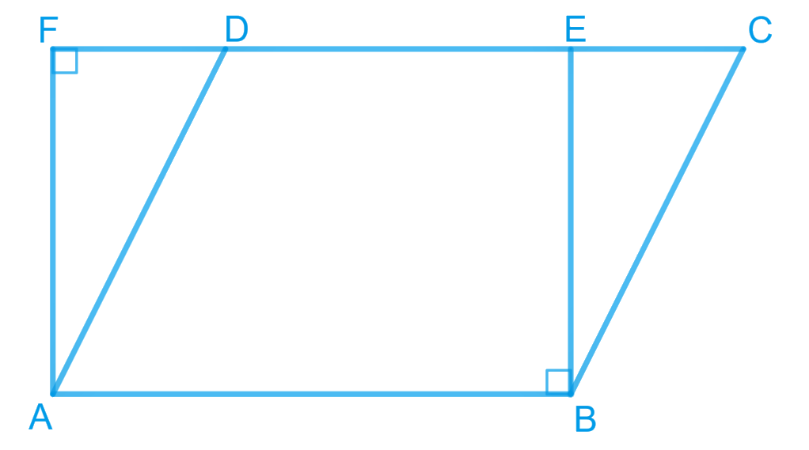Here, it can be observed that parallelogram $$ABCD$$ and rectangle $$ABEF$$ are between thesame parallels $$AB$$ and $$CF$$.

We know that opposite sides of a parallelogram or a rectangle are of equal lengths. Therefore,

\begin{align}&{AB}={EF} \text { (For rectangle) } \\ &{AB}={CD}(\text { For parallelogram }) \\ &\therefore {CD}={EF} \\ &\therefore {AB}+{CD}={AB}+{EF} \quad \ldots(1)\end{align}

Of all the line segments that can be drawn to a given line from a point not lying on it, the perpendicular line segment is the shortest.

$\therefore {AF}<{AD}$

And similarly, $$BE < BC$$

$\therefore {AF}+{BE}<{AD}+{BC}\quad \ldots(2)$

From Equations ($$1$$) and ($$2$$), we obtain

\begin{align} &{AB}+{EF}+{AF}+{BE} \\ & <{AD}+{BC}+{AB}+{CD} \end{align}

Perimeter of rectangle $$ABEF$$ $$<$$ Perimeter of parallelogram $$ABCD$$.

## Chapter 9 Ex.9.4 Question 2

In the following figure, $$D$$ and $$E$$ are two points on $$BC$$ such that $$BD = DE = EC$$.
Show that:

$$ar (ABD) = ar (ADE) = ar (AEC)$$.

### Solution

What is known?

$$D$$ and $$E$$ are two points on $$BC$$ such that  $$BD = DE = EC.$$

What is unknown?

How we can show that

$$\text{ar } \!\!( ABD ) = \!\text{ ar } \!\!( ADE ) = \text{ar } \!\! ( AEC ).$$

Reasoning:

First of all we can draw altitude which will help to find areas of all three triangles. As bases of all three triangles are equal so we can replace the value and we will find the required result.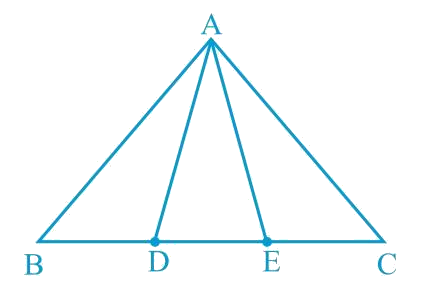Can you now answer the question that you have left in the ’Introduction’ of this chapter, whether the field of Budhia has been actually divided into three parts of equal area?

[Remark: Note that by taking $$BD = DE = EC$$, the triangle $$ABC$$ is divided into three triangles $$ABD$$, $$ADE$$ and $$AEC$$ of equal areas. In the same way, by dividing $$BC$$ into n equal parts and joining the points of division so obtained to the opposite vertex of $$BC$$, you can divide $$\Delta \rm{ABC}$$  into n triangles of equal areas.]

Steps:

Let us draw a line segment $$AL ⊥ BC$$.

##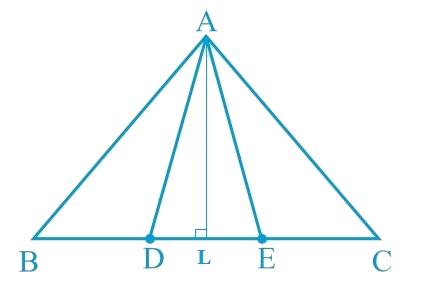We know that,

Area of a triangle $$= \frac{1}{2} \times$$ Base $$\times$$ Altitude

\begin{align} \text { Area }(\Delta {ADE})&=\frac{1}{2} \times {DE} \times {AL} \\ \text { Area }(\triangle {ABD})&=\frac{1}{2} \times {BD} \times {AL} \\ \text { Area }(\Delta {AEC})&=\frac{1}{2} \times {EC} \times {AL}\end{align}

It is given that

\begin{align}\frac{1}{2} \times DE \times AL &=\frac{1}{2} \times BD \times AL \\ &=\frac{1}{2} \times EC \times AL \\ \text { Area }(\Delta ADE)&=\text { Area }(\Delta ABD) \\ &=\text { Area }(\triangle AEC)\end{align}

It can be observed that Budhia has divided her field into  equal parts.

## Chapter 9 Ex.9.4 Question 3

In the following figure, $$ABCD$$, $$DCFE$$ and $$ABFE$$ are parallelograms. Show that ar ($$\Delta \rm{ADE}$$) $$=$$ ar ($$\Delta \rm{BCF}$$).

### Solution

What is known?

$$ABCD$$,$$DCFE$$ and $$ABFE$$ are parallelograms.

What is unknown?

How we can show that $$\text{ar }\left( \Delta \text{ADE} \right)\text{ = ar }\left( \Delta {BCF} \right)\text{.}$$

Reasoning:

We can see that sides of triangles $$ADE$$ and $$BCF$$ are also the opposite sides of the given parallelogram. Now we can show both the triangles congruent by SSS congruency. We know that congruent triangles have equal areas.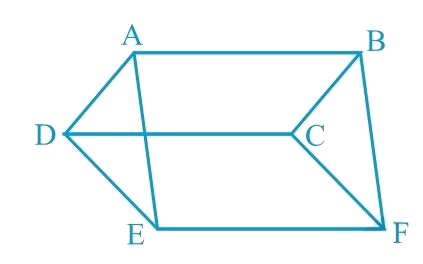Steps:

It is given that $$ABCD$$ is a parallelogram. We know that opposite sides of a parallelogram are equal.

$$\therefore \rm A D=B C \ldots(1)$$

Similarly, for parallelograms $$DCFE$$ and $$ABFE$$, it can be proved that

$$DE=CF$$.$$...(2)$$

And, $$EA = FB$$$$... (3)$$

In $$\Delta {ADE}$$ and $$\Delta {BCF}$$,

$$AD = BC$$ [Using equation ($$1$$)]

$$DE = CF$$ [Using equation ($$2$$)]

$$EA = FB$$ [Using equation ($$3$$)]

$$\therefore \Delta ADE \cong \Delta BCF\!\! \begin{pmatrix} \!\text { SSS} \!\\ \!\text{congruence } \!\\ \!\text{rule } \! \end{pmatrix}$$

The SSS Rule States that : If three sides of one triangle are equal to the three sides of another triangle, then the two triangles are congruent.

$\therefore \text { Area }(\Delta {ADE})=\text { Area }(\Delta {BCF})$

## Chapter 9 Ex.9.4 Question 4

In the following figure, $$ABCD$$ is parallelogram and $$BC$$ is produced to a point $$Q$$ such that $$AD = CQ$$. If $$AQ$$ intersect $$DC$$ at $$P$$, show that ar ($$\Delta BPC)$$ $$=$$ ar $$(\Delta DPQ)$$.

##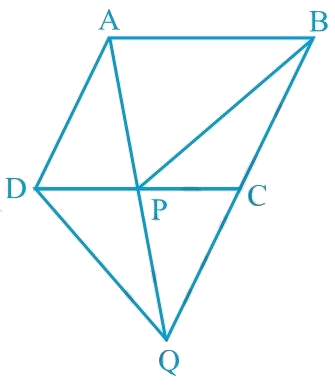[Hint: Join $$AC$$.]

#### ### Solution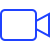Video Solution

What is known?

$$ABCD$$ is parallelogram and $$BC$$ is produced to a point $$Q$$ such that $$AD = CQ .$$  $$AQ$$ intersect $$DC$$ at $$P$$.

What is unknown?

How we can show that $$\text{ar}\left( \Delta BPC \right)=\text{ar}\left( \Delta DPQ \right).$$

Reasoning:

First of all, join $$AC$$. Now we can see that triangles $$APC$$ and $$BCP$$ are on same base and between parallel lines so areas will be equal. Similarly, triangles $$DQC$$ and $$ACQ$$ areas are equal and now subtract common area of triangle $$QPC$$ from both sides. Compare the result with first pair of triangles areas to get the required result.

#### Steps:

It is given that $$ABCD$$ is a parallelogram.

$$AD$$$$\parallel$$ $$BC$$ and $$AB$$ $$\parallel$$ $$DC$$ (Opposite sides of a parallelogram are parallel to each other) Join point $$A$$ to point $$C$$.

##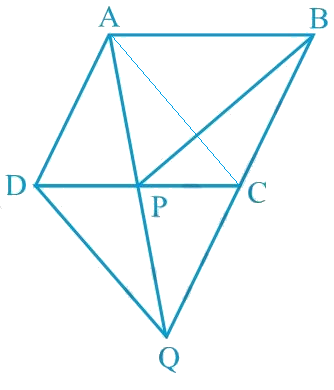Consider $$\Delta APC \text { and } \Delta BPC$$

$$\Delta APC \text { and } \Delta BPC$$ are lying on the same base $$PC$$ and between the same parallels $$PC$$ and $$AB$$.

According to Theorem $$9.2$$ : Two triangles on the same base (or equal bases) and between the same parallels are equal in area.

Therefore,

Area $$(\Delta APC)$$ = Area $$(\Delta BPC) \ldots(1)$$

In quadrilateral $$ACQD$$, it is given that

$$AD = CQ$$

Since $$ABCD$$ is a parallelogram,

$$AD\;|| \;BC$$ (Opposite sides of a parallelogram are parallel) $$CQ$$ is a line segment which is obtained when line segment $$BC$$ is produced.

$\therefore AD \; \| \; CQ$

We have,

$$AD = CQ$$ and $$AD\; ||\; CQ$$

Hence, $$ACQD$$ is a parallelogram.

Consider $$\Delta DCQ \text{and} \; \;\Delta ACQ$$

These are on the same base $$CQ$$ and between the same parallels $$CQ$$ and $$AD$$.

Therefore,

\begin{align} &\text { Area }(\Delta DCQ)=\text { Area }(\Delta ACQ) \end{align}

Therefore,

Area $$(\Delta DCQ)$$$$-$$ Area $$(\Delta PQC)$$= Area $$(\Delta ACQ)$$ - Area $$(\Delta PQC)$$

Subtracting Area $$(\Delta PQC)$$ on both the sides .

Therefore,

Area $$(\Delta DPQ)$$ = Area $$(\Delta APC) \ldots(2)$$

From equations (1) and (2), we obtain

$\text { Area }(\Delta BPC)=\text { Area }(\Delta DPQ)$

## Chapter 9 Ex.9.4 Question 5

In the following figure, $$ABC$$ and $$BDE$$ are two equilateral triangles such that $$D$$ is the mid-point of $$BC$$. If $$AE$$ intersects $$BC$$ at $$F$$, show that

(i) \begin{align} \operatorname{ar}({BDE})=\frac{1}{4} \operatorname{ar}({ABC})\end{align}

(ii) \begin{align} \operatorname{ar}({BDE})=\frac{1}{2} \operatorname{ar}({BAE})\end{align}

(iii) $$\operatorname{ar}({ABC})=2 \text { ar }({BEC})$$

(iv) $$\operatorname{ar}({BFE})= \text { ar }({AFD})$$

(v) $$\operatorname{ar}({BFE})= 2 \text { ar }({FED})$$

(vi) \begin{align} \operatorname{ar}({FED})= \frac{1}{8} \text { ar }({AFC})\end{align}

### Solution

What is known?

$$ABC$$ and $$BDE$$ are two equilateral triangles such that $$D$$ is the mid-point of $$BC. AE$$ intersects $$BC$$ at $$F$$.

What is unknown?

How we can show that

(i) \begin{align} \operatorname{ar}({BDE})=\frac{1}{4} \operatorname{ar}({ABC})\end{align}

(ii) \begin{align} \operatorname{ar}({BDE})=\frac{1}{2} \operatorname{ar}({BAE})\end{align}

(iii) $$\operatorname{ar}({ABC})=2 \text { ar }({BEC})$$

(iv) $$\operatorname{ar}({BFE})= \text { ar }({AFD})$$

(v) $$\operatorname{ar}({BFE})= 2 \text { ar }({FED})$$

(vi) \begin{align} \operatorname{ar}({FED})= \frac{1}{8} \text { ar }({AFC})\end{align}

Reasoning:

For the first part let $$G$$ and $$H$$ be the mid-points of side $$AB$$ and $$AC$$ and now we can use mid-point theorem. For remaining parts join vertices $$E$$ to $$C$$ and $$A$$ to $$D$$. Now we use the facts that median divides a triangle area in to two triangles of equal areas, If two triangles are on same base and between same pair of parallel lines then areas will be equal of both the triangles.

##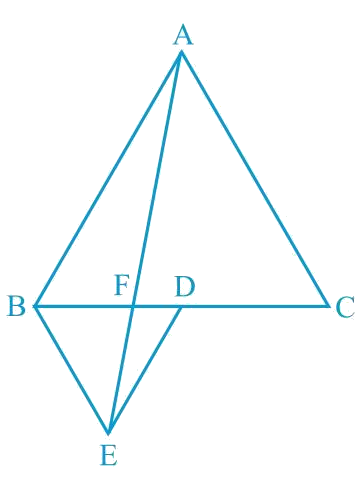[Hint: Join $$EC$$ and $$AD$$. Show that $$BE$$ $$\|$$ $$AC$$ and $$DE$$ $$\|$$ $$AB$$ etc.]

#### Steps:

(i) Let $$G$$ and $$H$$ be the mid-points of side $$AB$$ and $$AC$$ respectively.

Line segment $$GH$$ is joining the mid-points and is parallel to third side. Therefore, $$GH$$ will be half of the length of $$BC$$ (mid-point theorem).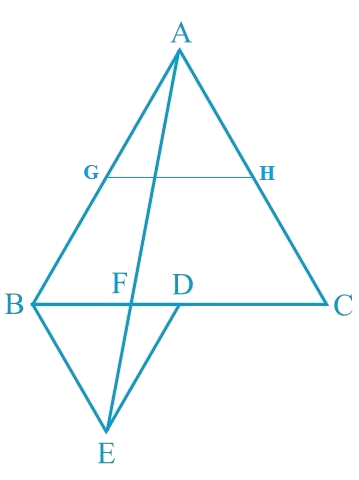\begin{align}&\therefore {GH}=\frac{1}{2} BC \text{ and} \, {GH} \| {BD} \\ &\therefore {GH}={BD}={DC} \text { and } \\ \\ &{GH} \| {BD}({D} \text { is the mid-point of } {BC})\end{align}

Similarly,

• $$GD = HC = HA$$
• $$HD = AG = BG$$

Therefore, clearly $$\Delta$$$$ABC$$ is divided into $$4$$ equal equilateral triangles viz $$\Delta$$$$BGD$$, $$\Delta$$$$AGH$$, $$\Delta$$$$DHC$$ and $$\Delta$$ $$GHD$$

In other words,

\begin{align}\text { Area }(\Delta {BGD})=\frac{1}{4} \text { Area }(\Delta {ABC}) \end{align}

Now consider $$\Delta {BDG} \text { and } \Delta {BDE}$$

$$BD = BD$$ (Common base)

As both triangles are equilateral triangles, we can say

$$BG = BE$$

$$DG = DE$$

Therefore,

$$\Delta {BDG} \cong \Delta {BDE}$$ [By SSS congruency]

The SSS rule states that: If three sides of one triangle are equal to three sides of another triangle, then the triangles are congruent.

Thus, $$\text { area }(\Delta {BDG})=\text { area }(\Delta {BDE})$$

\begin{align} \therefore \operatorname{ar}(\Delta {BDE})=\frac{1}{4} \operatorname{ar}(\Delta {ABC}) \end{align}

Hence proved

##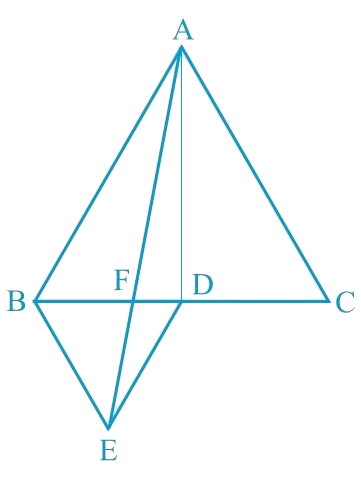(ii) Area $$(\Delta {BDE})=$$ Area $$(\triangle {AED})$$  (Common base $$DE$$ and $$DE \| AB$$)

Area $$(\Delta {BDE})\, -$$ Area $$(\Delta {FED})\,=$$ Area $$(\Delta {AED})\, -$$ Area $$(\Delta {FED})$$

Area $$(\Delta {BEF})=$$ Area $$(\Delta {AFD}) \ldots (1)$$

Now,

Area $$(\Delta {ABD})=$$Area $$(\triangle {ABF})+$$ Area $$(\triangle {AFD})$$

Area $$( \Delta ABD ) =$$ Area $$( \Delta ABF) +$$ Area $$( \Delta BEF )$$ [ From equation ($$1$$) ]

Area $$(\Delta {ABD})=$$ Area $$(\Delta {ABE})$$  $$\ldots (2)$$

$$AD$$ is the median in $$\Delta {ABC}$$.

\begin{align} \text{ar}(\Delta {ABD}) &=\frac{1}{2} \text{ar}(\Delta {ABC}) \\ &=\frac{4}{2} \text{ar}(\Delta {BDE}) \\ & \quad \begin{pmatrix} \text{As }\\ \text{proved} \\ \text{ earlier } \end{pmatrix} \\ \text{ar}(\Delta {ABD})&=2 \text{ar}(\Delta {BDE}) \cdots (3) \end{align}

From Equation (2) and (3), we obtain

\begin{align} 2 \operatorname{ar}(\Delta {BDE})=\operatorname{ar}(\Delta {ABE}) \\ \operatorname{ar}({BDE})=\frac{1}{2} \text { ar }({BAE})\end{align}

(iii)

ar $$(\Delta {ABE})=$$ ar $$(\Delta {BEC})$$ (Common base $$BE$$ and $$BE \| AC$$ )

ar $$(\Delta ABF) +$$ar $$(\Delta BEF) =$$ ar $$(\Delta BEC)$$

Using equation $$(1)$$ we obtain

\begin{align}& \!\!\begin{pmatrix} \text{ar}(\Delta {ABF})\\+\operatorname{ar}(\Delta {AFD}) \end{pmatrix} \!\!=\operatorname{ar}(\Delta {BEC}) \\ &\operatorname{ar}(\Delta {ABD})=\operatorname{ar}(\Delta {BEC}) \\ &\frac{1}{2} \operatorname{ar}(\Delta {ABC})=\operatorname{ar}(\Delta {BEC}) \\ &\operatorname{ar}(\Delta {ABC})=2 \operatorname{ar}(\Delta {BEC})\end{align}

(iv) It is seen that $$\Delta BDE \text { and ar } \Delta {AED}$$ lie on the same base (DE) and between the parallels $$DE$$ and $$AB$$.

\begin{align} &\therefore \operatorname{ar}(\Delta {BDE})=\operatorname{ar}(\Delta {AED}) \\ \\&\therefore \operatorname{ar}(\Delta {BDE})-\operatorname{ar}(\Delta {FED}) \\ & \quad=\operatorname{ar}(\Delta {AED})-\text{ar}(\Delta {FED}) \end{align}

Subtracting ar $$(\Delta {FED})$$ on both the sides

$\therefore \operatorname{ar}(\Delta {BFE})=\operatorname{ar}(\Delta {AFD})$

(v) Let h be the height of vertex $$E$$, corresponding to the side $$BD$$ in $$\Delta BDE$$. Let $$H$$ be the height of vertex $$A$$, corresponding to the side $$BC$$ in $$\Delta ABC$$.

In (i), it was shown that

\begin{align} \operatorname{ar}({BDE})=\frac{1}{4} \operatorname{ar}({ABC})\end{align}

In (iv), it was shown that

ar $$(\Delta {BFE})=\operatorname{ar}(\Delta {AFD})$$.

$\therefore {\rm{ ar (}}\Delta BFE) = \text{ar} (\Delta AFD)$

${\rm{ = 2 ar (}}\Delta FED)$

Hence,

(vi)

\begin{align} & {\rm{ar }}\left( {\Delta AFC} \right) \\ &= {\rm{ar }}\left( {\Delta AFD} \right){\rm{ + ar }}\left( {\Delta ADC} \right) \\ &= \begin{pmatrix} 2 {\rm{ar }}\left( {\Delta FED} \right) \\ + \frac{1}{2}{\rm{ ar }}\left( {\Delta ABC} \right) \\ {\rm{ [using }}\left( {\rm{v}} \right)] \end{pmatrix}\end{align}

\begin{align} &= \begin{pmatrix} 2 \text{ar} \left( {\Delta FED} \right) \\+ \frac{1}{2} \left[ 4 \times {\rm{ar }}\left( {\Delta BDE} \right) \right] \end{pmatrix} \\ & \quad \left[ {{\text{Using result of part }}\left( {\rm{i}} \right)} \right] \end{align}

\begin{align} &= 2 \text{ar} \left( {\Delta FED} \right){\rm{ + 2 ar }}\left( {\Delta BDE} \right) \\ &= 2 \text{ar} \left( {\Delta FED} \right){\rm{ + 2 ar }}\left( {\Delta AED} \right)\\&= \begin{bmatrix} \Delta BDE \;\text{and} \;\Delta AED \\ \text{are on the same base} \\ \text{ and between same} \\ \text{ parallels}\end{bmatrix} \end{align}

\begin{align} &= \begin{pmatrix} 2 \text{ar}\left( \Delta FED \right) \\+ 2 \begin{bmatrix} {\rm{ar }}\left( \Delta AFD \right) \\ + {\rm{ ar }}\left( \Delta FED \right) \end{bmatrix} \end{pmatrix} \\ &= \begin{pmatrix}2 \text{ar} \left( {\Delta FED} \right) \\+ 2 {\rm{ ar }}\left( {\Delta AFD} \right) \\+ 2 {\rm{ ar }}\left( {\Delta FED} \right) \\ \left[ {{\rm{Using }}\left( {{\rm{viii}}} \right)} \right] \\ \end{pmatrix} \\ &= 4 \text{ar} \left( {\Delta {{FED}}} \right){{ + 4 ar }}\left( {\Delta {{FED}}} \right)\\&\Rightarrow {\rm{ar }}\left( {\Delta {{AFC}}} \right)\rm = 8 ar \left( {\Delta {{FED}}} \right)\\&\Rightarrow {\rm{ar }}\left( {\Delta {{FED}}} \right){\rm = }\frac{1}{8}{\rm{ ar }}\left( {\Delta {{AFC}}} \right) \end{align}

## Chapter 9 Ex.9.4 Question 6

Diagonals $$AC$$ and $$BD$$ of a quadrilateral $$ABCD$$ intersect each other at $$P$$. Show that

\begin{align}&{\rm{ ar }}\left( {\Delta APB} \right) \times {\rm{ ar }}\left( {\Delta CPD} \right) \\ &= {\rm{ ar }}\left( {\Delta APD} \right) \times {\rm{ ar }}\left( {\Delta BPC}\right)\end{align}

[Hint: From $$A$$ and $$C$$, draw perpendiculars to $$BD$$]

### Solution

What is known?

A quadrilateral ABCD, in which diagonals AC and BD intersect each other at point P.

What is unknown?

To Prove:

\begin{align}&{\rm{ ar }}\left( {\Delta APB} \right) \times {\rm{ ar }}\left( {\Delta CPD} \right) \\ &= {\rm{ ar }}\left( {\Delta APD} \right) \times {\rm{ ar }}\left( {\Delta BPC}\right)\end{align}

Reasoning:

From A, draw $$AM\bot BD$$ and from C, draw $$CN\bot BD.$$ Now we can find area of triangles to get the required result.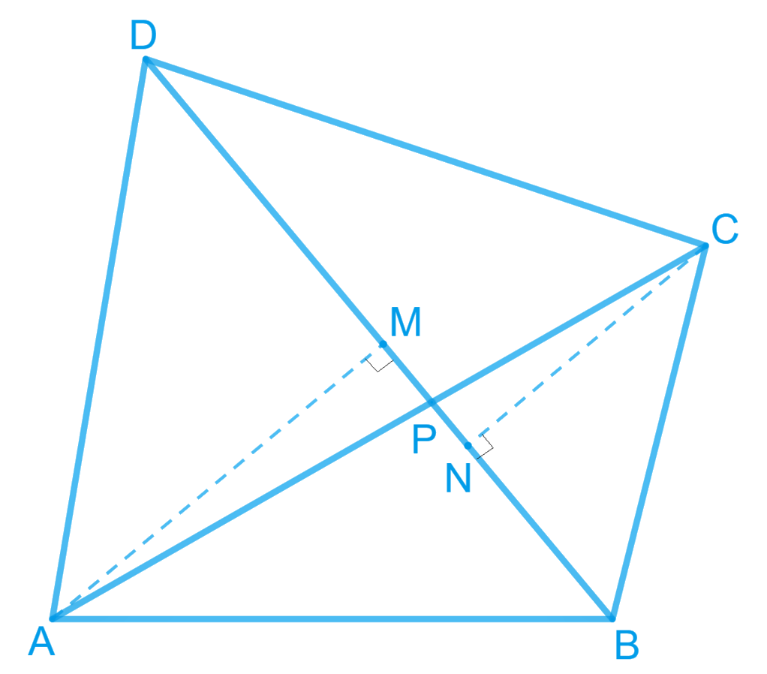Steps:

Proof :

\begin{align} \text{ ar} (\Delta ABP) & \!\!= \!\frac{1}{2} \!\!\times PB \!\times \! AM...\text{(i)} \\ \text{ar} (\Delta APD) & \!\!=\!\frac{1}{2} \!\times \! PD \! \times \! AM...\text{(ii)} \end{align}

Dividing eq. (ii) by (i), we get,

\begin{align} \frac{\text{ar} (\Delta \text{APD)}}{\text{ar} (\Delta ABP)} & =\frac{\frac{1}{2} \times PD\times AM}{\frac{1}{2}\times PB\times AM} \\ \Rightarrow \frac{\text{ar}(\Delta APD)}{\text{ar}(\Delta ABP)}&=\frac{PD}{PB}.....(\text{iii}) \\ \end{align}

Similarly,

\begin{align} & \frac{\text{ar}(\Delta CDP)}{\text{ar}(\Delta BPC)}=\frac{PD}{PB}....(\text{iv}) \end{align}

From eq . (iii) and (iv), we get

\begin{align} & \frac{\text{ar}(\Delta APD)}{\text{ar}(\Delta ABP)}=\frac{\text{ar}(\Delta CDP)}{\text{ar} (\Delta BPC)} \\ & \Rightarrow \text{ar} (\Delta APD)\times \text{ar}(\Delta BPC) \\ & \quad =\text{ar}(\Delta ABP) \times \text{ar} (\Delta CDP) \end{align}

Hence proved.

## Chapter 9 Ex.9.4 Question 7

$$P$$ and $$Q$$ are respectively the mid-points of sides $$AB$$ and $$BC$$ of a triangle $$ABC$$ and $$R$$ is the mid-point of $$AP$$, show that

(i) \begin{align}{\rm{ar}} (PRQ) = \,\,\frac{{\rm{1}}}{{\rm{2}}}{\rm{ ar}} (ARC)\end{align}

(ii) \begin{align}{\rm{ar }} (RQC) =\,\,\frac{3}{8}{\rm{ ar}}(ABC)\end{align}

(iii) \begin{align}{\rm{ar }} (PBQ) = {\rm{ar }} (ARC)\end{align}

### Solution

#### Steps:

(i) $$PC$$ is the median of $$\Delta ABC.$$

\begin{align}\therefore \operatorname{ar}(\Delta BPC)=\operatorname{ar}(\Delta APC) \ldots .(\mathrm{i})\end{align}

$$RC$$ is the median of $$\Delta APC.$$

\begin{align}\therefore \operatorname{ar}(\Delta ARC)= \!\frac{1}{2} \!\operatorname{ar}(\Delta APC)\! \ldots (\mathrm{ii})\end{align}

[Median divides the triangle into two triangles of equal area]

$$PQ$$ is the median of $$\Delta BPC.$$

####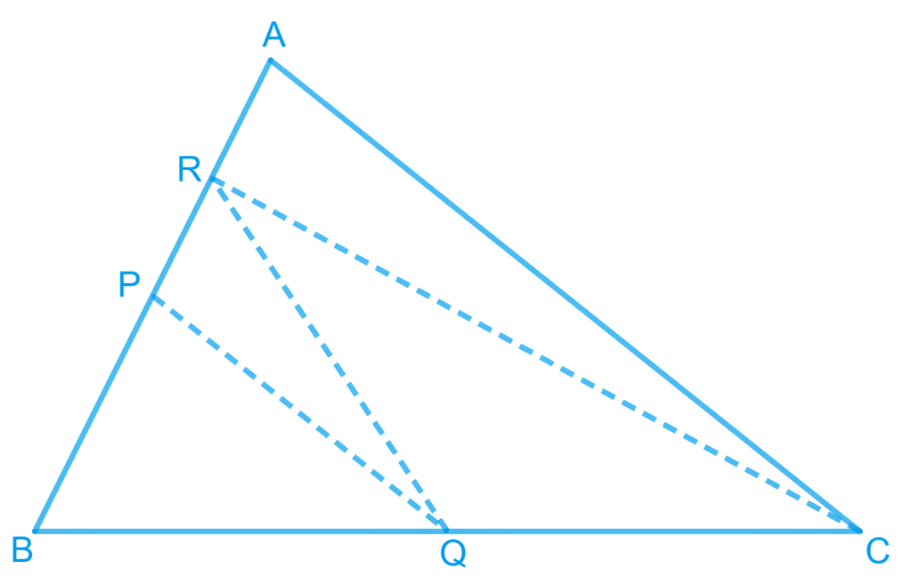\begin{align}\therefore \operatorname{ar}(\Delta PQC) \!\!=\! \frac{1}{2}\operatorname{ar}(\Delta BPC) \!\!\ldots (\mathrm{iii})\end{align}

From eq. (i) and (iii), we get,

\begin{align} \text{ar}(\Delta PQC)= \frac{1}{2}\operatorname{ar}(\Delta APC) \ldots .(\mathrm{iv})\end{align}

From eq. (ii) and (iv), we get,

\begin{align} \text{ar}(\Delta PQC)= \operatorname{ar}(\Delta ARC) \ldots .(\mathrm{v})\end{align}

We are given that $$P$$ and $$Q$$ are the mid-points of $$AB$$ and $$BC$$ respectively.

\begin{align} \therefore PQ \| AC \text { and } PQ=\frac{1}{2} AC \end{align}

\begin{align} \Rightarrow & \operatorname{ar}(\Delta APQ) \\ &= \operatorname{ar}(\Delta PQC) \ldots .(\mathrm{vi})\end{align}

[triangles between the same parallel lines are equal in area]

From eq. (v) and (vi), we get

$$\operatorname{ar}(\Delta APQ)= \operatorname{ar}(\Delta ARC) \ldots (\mathrm{vii})$$

$$R$$ is the mid-point of $$AP$$. Therefore, $$RQ$$ is the median of $$\Delta APQ.$$

\begin{align}\therefore\; &\operatorname{ar}(\Delta PRQ) \\ &=\frac{1}{2} \operatorname{ar}(\Delta APQ) \ldots .(\mathrm{viii}) \end{align}

From (vii) and (viii), we get,

\begin{align}\therefore \operatorname{ar}(\Delta PRQ)=\frac{1}{2} \operatorname{ar}(\Delta ARC)\end{align}

(ii) $$PQ$$ is the median of $$\Delta BPC.$$

\begin{align}\therefore \; & {\rm{ar}}\,\left( {\Delta PQC} \right) \\ & = \frac{1}{2}\,{\rm{ar}} (\Delta BPC) \\ &= \frac{1}{2} \times \frac{1}{2} {\rm{ar }}\left( {\Delta ABC} \right)....\rm(ix)\end{align}

Also,

$${\text{ar}}\left( {\Delta PRC} \right) = \begin{pmatrix} \frac{1}{2} {\rm{ar}} (\Delta APC) \\ {\text{ [Using (iv)]}} \end{pmatrix}$$

\begin{align} \Rightarrow \, & {\rm{ar}}\left( {\Delta PRC} \right) \\ &= \frac{1}{2} \times \frac{1}{2}{\rm{ar}}\,(\Delta ABC) \\ &= \frac{1}{4}{\rm{ar}}\,(\Delta ABC)...\rm(x) \end{align}

Adding eq. (ix) and (x), we get,

\begin{align} & \operatorname{ar}(\Delta PQC)+\operatorname{ar}(\Delta PRC) \\ & = \left(\frac{1}{4}+\frac{1}{4}\right) \operatorname{ar}(\Delta ABC) \end{align}

\begin{align}\Rightarrow \, & {\rm{ar }}\left( PQCR \right) \\ &= \frac{1}{2}{\rm{ar}}\left( {\Delta ABC} \right)...\left( {{\rm{xi}}} \right) \end{align}

Subtracting ar $$(\Delta PRQ)$$ from the both sides,

\begin{align} &{\rm{ ar}}(\Delta PQCR )-\operatorname{ar}(\Delta PRQ) \\ & \quad =\frac{1}{2} \operatorname{ar}(\Delta ABC)-\operatorname{ar}(\Delta PRQ) \end{align}

\begin{align}&\Rightarrow {\rm{ar}}\,\Delta RQC) \\ & \quad = \begin{pmatrix} \frac{1}{2} {\rm{ar}}( \Delta ABC) \\ - \frac{1}{2}{\rm{ar}}(\Delta ARC) \\ \text{[Using result (i)]} \end{pmatrix}\end{align}

\begin{align} &\Rightarrow {\rm{ar}}( \Delta ARC) \\ & \quad=\begin{pmatrix} \frac{1}{2}{\rm{ ar}} (\Delta ABC) \\ - \frac{1}{2} \times \frac{1}{2}{\rm{ar}}(\Delta APC) \end{pmatrix} \\ &\Rightarrow {\rm{ar}}(\Delta RQC) \\ & \quad =\begin{pmatrix} \frac{1}{2}{\rm{ ar}}(\Delta ABC) \\ - \frac{1}{4} {\rm{ar}}(\Delta APC)\end{pmatrix} \end{align}

\begin{align} \Rightarrow \;& \text{ar}(\Delta RQC) \\ &= \begin{pmatrix}\frac{1}{2} \operatorname{ar}(\Delta ABC) - \\ \frac{1}{4} \times \frac{1}{2} \operatorname{ar}(\Delta ABC) \\ \begin{bmatrix} PC \text { is median of }\\ \Delta ABC\end{bmatrix} \end{pmatrix} \end{align}

\begin{align}\Rightarrow \,&\text{ar}(\Delta RQC) \\ &= \begin{pmatrix} \frac{1}{2} \operatorname{ar}(\Delta ABC) \\ -\frac{1}{8} \operatorname{ar}(\Delta ABC) \end{pmatrix} \end{align}

\begin{align}\Rightarrow \, & \text{ar}(\Delta RQC) \\ &= \begin{pmatrix} \left(\frac{1}{2}-\frac{1}{8}\right) \\ \times \operatorname{ar}(\Delta ABC) \end{pmatrix} \end{align}

\begin{align}\Rightarrow \, &\text{ar}(\Delta RQC) \\ &=\frac{3}{8} \times \operatorname{ar}(\Delta ABC) \end{align}

(iii)

\begin{align} {\rm{ar }}\left( {\Delta PRQ} \right) &= \frac{1}{2}{\rm{ar }}\left( {\Delta ARC} \right)\, \\ & \quad [\rm Using\,{\rm{result (i)}}] \end{align}
\begin{align} \Rightarrow \, & 2{\rm{ar}}\left( {\Delta PRQ} \right) \\ &= {\rm{ar}}\left( {\Delta ARC} \right)...\rm(xii)\end{align}

\begin{align}\text{ar}(\Delta PRQ) &=\frac{1}{2} \text{ ar }(\Delta APQ) \\ & \;\; \begin{bmatrix} RQ \text{ is the}\\ \text{ median of} \\ \Delta APQ \end{bmatrix} \!\!\dots \text{(xiii)}\end{align}

But,

\begin{align} & \text{ ar } \left( {\Delta APQ} \right) \\ & = {\rm{ ar }}\left( {\Delta PQC} \right) \\ & \quad \begin{bmatrix} \text{Using reason } \\ \text{of eq}.( {\rm{vi}} ) \end{bmatrix}...\left( {\rm{xiv}} \right) \end{align}

From eq. ($$\rm{xiii}$$) and ($$\rm{xiv}$$), we get,

\begin{align}\operatorname{ar}(\Delta PRQ)=\frac{1}{2} \operatorname{ar}(\Delta PQC) \ldots(\mathrm{xv})\end{align}

But,

\begin{align}\text{ ar} \left( {\Delta BPQ} \right) &= {\rm{ ar}}\left( {\Delta PQC} \right) \\ & \begin{bmatrix} PQ \text{ is the} \\ \text{ median of } \\ \Delta BPC \end{bmatrix}\!\!...\left( {\rm{xvi}} \right) \end{align}

From eq. ($$\rm{xv}$$) and ($$\rm{xvi}$$), we get,

\begin{align} &\text{ar} (\Delta PRQ) \\ &=\frac{1}{2} \operatorname{ar}(\Delta BPQ) \!\! \ldots\rm (xvii)\end{align}

Now from ($$\rm{xii}$$) and ($$\rm{xvii}$$), we get,

\begin{align} 2 \times \frac{1}{2} \text{ar} (\Delta BPQ) & = {\rm{ar}}(ARC) \\ \Rightarrow \quad \quad {\rm{ar}} (\Delta BPQ) & = {\rm{ar(}}\Delta ARC) \end{align}

## Chapter 9 Ex.9.4 Question 8

In the following figure, $$ABC$$ is a right triangle right angled at $$A$$. $$BCED$$, $$ACFG$$ and $$ABMN$$ are squares on the sides $$BC$$, $$CA$$ and $$AB$$ respectively. Line segment $$AX ⊥ DE$$ meets $$BC$$ at $$Y$$.

##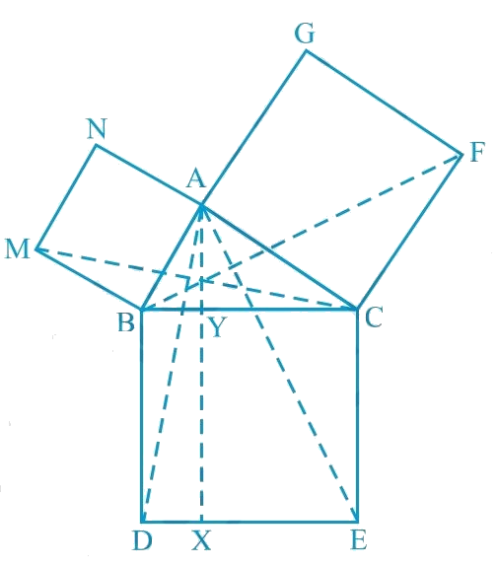Show that:

(i) \begin{align}\Delta MBC \cong \Delta ABD \end{align}

(ii) \begin{align}\operatorname{ar}(BYXD)=2 \operatorname{ar}(MBC)\end{align}

(iii) \begin{align}\operatorname{ar}(BYXD)=2 \operatorname{ar}(ABMN)\end{align}

(iv) \begin{align}\Delta FCB \cong \Delta ACE \end{align}

(v) \begin{align}\operatorname{ar}(CYXE)=2 \operatorname{ar}(FCB)\end{align}

(vi) \begin{align}\operatorname{ar}(CYXE )= \operatorname{ar}(ACFG)\end{align}

(vii) \begin{align} \begin{bmatrix} \operatorname{ar}(BCED) \\ \begin{Bmatrix}= {\rm{ar}} (ABMN)\\ + \operatorname{ar}(ACFG)\end{Bmatrix} \end{bmatrix} \end{align}

Note: Result (vii) is the famous Theorem of Pythagoras. You shall learn a simpler proof of this theorem in class X.

### Solution

#### Steps:

(i) We know that each angle in a square measures $$90^\circ$$.

Hence,

$\angle ABM =\angle DBC=90^{\circ}$

\begin{align} &\therefore \angle ABM+\angle ABC \\& \qquad=\angle DBC+\angle ABC \\& \therefore \angle MBC=\angle ABD \end{align}

In $$\Delta MBC$$ and $$\Delta ABD ,$$

$$\angle MBC$$ $$=$$ $$\angle ABD$$ (Proved above)

$$MB = AB$$ (Sides of square $$ABMN$$)

$$BC = BD$$ (Sides of square $$BCED$$)

\begin{align}\therefore \Delta MBC & \cong \Delta ABD \\ &\begin{pmatrix} \text { SAS } \\ \text{congruence} \\ \text{ rule } \end{pmatrix}\end{align}

(ii) We have

\begin{align} &\Delta MBC \cong \Delta ABD \\ \therefore \, & \operatorname{ar}(\Delta MBC) \\ & = \text{ar}(\Delta ABD) \!\! \ldots(1)\end{align}

It is given that $$AX ⊥ DE$$ and $$BD ⊥ DE$$ (Adjacent sides of square $$BDEC$$)

$$\therefore BD\; \| \; \mathrm{AX}$$ (Two lines perpendicular to the same line are parallel to each other.)

$$\Delta ABD$$ and parallelogram $$BYXD$$ are on the same base $$BD$$ and between the same parallels $$BD$$ and $$AX$$.

\begin{align} & \text {Area}(\Delta BYXD) \\ &=2 \text { Area }(\Delta MBC) \\ & \quad \begin{bmatrix} \text { Using} \\ \text{ equation }(1) \end{bmatrix} \ldots(2) \end{align}

(iii)  $$\Delta MBC$$ and parallelogram $$ABMN$$ are lying on the same base $$MB$$ and between same parallels $$MB$$ and $$NC$$.

\begin{align} &2 \operatorname{ar}(\Delta MBC) \\ & =\operatorname{ar}(ABMN) \\ \\ & \text { ar }(\Delta BYXD) \\ & =\operatorname{ar}(ABMN) \\ & \quad \begin{bmatrix} \text { Using } \\ \text{equation }(2) \end{bmatrix} \ldots(3)\end{align}

(iv) We know that each angle of a square is $$90^\circ$$.

\begin{align}&\therefore \angle FCA =\angle BCE =90^{\circ} \\& \therefore \angle FCA +\angle ACB \\ & \quad =\!\! \begin{pmatrix} \angle BCE \\ +\angle ACB \end{pmatrix} \end{align}

Adding $$\angle$$$$ACB$$ on both the sides

$$\therefore \angle FCB=\angle ACE$$

In $$\Delta FCB$$ and $$\Delta ACE$$,

\begin{align} \angle FCB &= \angle AC \\ & \quad \begin{pmatrix} \text{Sides of } \\ \text{square} \\ ACFG \end{pmatrix} \end{align}

$$CB = CE$$ (Sides of square $$BCED$$)

\begin{align} \Delta FCB & \cong \triangle ACE \\ & \quad \begin{pmatrix} \text { SAS } \\ \text{congruence} \\ \text{ rule } \end{pmatrix} \end{align}

SAS congruence rule- if two sides and the included angle of one triangle are equal to the two sides and the included angle of the other triangle then the triangles are congruent.

(v) It is given that $$AX ⊥ DE$$ and $$CE ⊥ DE$$ (Adjacent sides of square $$BDEC$$)

Hence, $$CE\;|| \;AX$$ (Two lines perpendicular to the same line are parallel to each other)

Consider $$\Delta ACE$$ and parallelogram $$CYXE$$

$$\Delta ACE$$ and parallelogram $$CYXE$$ are on the same base $$CE$$ and between the same parallels $$CE$$ and $$AX$$.

\begin{align} \therefore \, & \text{ ar }(\Delta CYXE) \\ & = 2 \text { ar }(\Delta ACE) \ldots(4) \end{align}

$$\therefore \Delta FCB \cong \triangle ACE$$

$$\operatorname{ar}(\Delta FCB) \cong \operatorname{ar}(\Delta ACE) \ldots(5)$$

On comparing equations (4) and (5),

we obtain

$$\text{ar} (CYXE) = 2 \text{ar} \, (\Delta FCB)$$ ... (6)

(vi) Consider $$\Delta FCB$$ and parallelogram $$ACFG$$

$$\Delta FCB$$ and parallelogram $$ACFG$$ are lying on the same base $$CF$$ and between the same parallels $$CF$$ and $$BG$$.

\begin{align}\therefore \operatorname{ar} (ACFG) & =2 \operatorname{ar}(\Delta FCB) \\ \therefore \operatorname{ar} (ACFG) &=\operatorname{ar}(CYXE) \\ & \quad \begin{bmatrix}\text { Using} \! \\ \! \text{ equation } \! \\ (6) \!\end{bmatrix} \!\!\ldots(7) \end{align}

(vii) From the figure, it is evident that

\begin{align} &{\rm{ar}} (\Delta BCED) \\ &= \begin{bmatrix} \operatorname{ar}(\Delta BYXD) \\+\mathrm{ar}(CYXE) \end{bmatrix} \end{align}

\begin{align} \therefore \, & \operatorname{ar}(\Delta BCED) \\ &=\operatorname{ar}(ABMN)+\operatorname{ar}(ACFG) \\ & \quad \begin{bmatrix} \text { Using equations } \\ (3) \text { and }(7) \end{bmatrix} . \end{align}

Related Sections
Related Sections
Instant doubt clearing with Cuemath Advanced Math Program